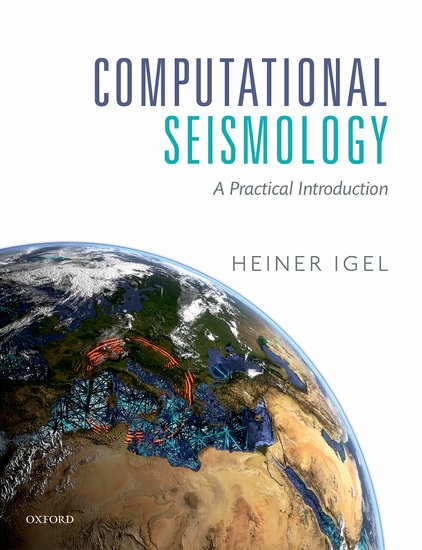Computational Seismology
Finite Differences - Advection Diffusion Reaction EquationThis notebook is part of the supplementary material to Computational Seismology: A Practical Introduction, Oxford University Press, 2016.

##### Authors:¶

This exercise covers the following aspects:

• Solving the advection-diffusion-reaction equation with different schemes of finite difference method
• Investigation of different numerical solutions and finding the stable one

## Basic Equations¶

Please refer to the Exercise 4.20 on Page 105 from the book.

The advection-diffusion-reaction equation is given by

$$\partial_t C(x,t) = k \partial_x^2 C(x,t) + v \partial_x C(x,t) - R(x)C(x,t) + P(x)$$

Where,

$C(x,t)$ is the concentration,

$k$ is the diffusivity,

$R(x)$ is the reactivity,

$P(x)$ is the source, and

$v$ is the advection velocity.

We replace the derivatives as follows

$$\partial_x C(x,t) \ \approx \ \frac{C(x + \mathrm{d}x, t) - C(x -\mathrm{d}x,t)} {2 \mathrm{d}x}$$$$\partial_x^2 C(x,t) \ \approx \ \frac{C(x + \mathrm{d}x, t) - 2C(x,t) + C(x - \mathrm{d}x, t)}{\mathrm{d}x^2}$$$$\partial_t C(x,t) \ \approx \ \frac{C(x, t + \mathrm{d}t) - C(x,t)}{ \mathrm{d}t}$$

The R.H.S. of the advection-diffusion-reaction becomes

$$R.H.S. = dc = k \bigg[ \frac{C(x + \mathrm{d}x) -2 C(x) + C(x - \mathrm{d}x)}{\mathrm{d} x^2} \bigg] + v \bigg[ \frac{C(x + \mathrm{d}x) - C(x -\mathrm{d}x)}{2dx} \bigg] - RC + P$$

Now, solving for $C(x, t + \mathrm{d}t)$ the extrapolation scheme is

$$C(x, t + \mathrm{d}t) = dc \mathrm{d}t + C(t) = k \mathrm{d}t \bigg[ \frac{C(x + \mathrm{d}x) -2 C(x) + C(x - \mathrm{d}x)}{\mathrm{d} x^2} \bigg] + v \mathrm{d}t \bigg[ \frac{C(x + \mathrm{d}x) - C(x -\mathrm{d}x)}{2dx} \bigg] - RC \mathrm{d}t + P \mathrm{d}t + C(t)$$

Note that we used centered finite-difference (in velocity term) scheme.

### Exercise¶

First understand the following codes, run the simulation and interpret the solution (plot).

Then, implement the forward and the backward (in velocity term) finite-difference schemes. Investigate different numerical solutions. Which scheme is stable?

Message: Once you become familiar with all the codes below you can go to the Cell tab on the toolbar and click Run All.

In :
# Configuration step (Please run it before the simulation code!)

import numpy as np
import matplotlib
# Show Plot in The Notebook
matplotlib.use("nbagg")
import matplotlib.pyplot as plt
matplotlib.rcParams['figure.facecolor'] = 'w'         # remove grey background
from mpl_toolkits.mplot3d import Axes3D               # for 3D plot

In :
# Initialization of parameters

k = 3*np.exp(4)                                       # eddie mixing (diffusivity) in km^2/year
v = 1500.                                             # velocity km/year
p0 = 0.01                                             # production rate in mol/year
r = 2*np.exp(-3)                                      # removal rate (reactivity) in mol/year

# Choose among "centered", "forward" or "backward" finite-difference scheme.
fd_type = "forward"
#fd_type = "centered"
#fd_type = "backward"

# Space discretization
nt = 300                                              # number of time steps
nx = 100                                              # space dimension

phi = 2*np.pi/(nx-1)*np.arange(0,nx)
xc = np.cos(phi)                                      # for visualization of eddie like circular plot
yc = np.sin(phi)                                      # for visualization of eddie like circular plot

dt = 0.01                                             # years
dx = 1000                                             # km
isnap = 5                                             # snapshot frequency for visualization

# Initialization of variables
c = np.zeros(nx)                                      # concentration
dc =np.zeros(nx)                                      # R.H.S. of equation
p = np.zeros(nx)                                      # source
p[int((nx-1)/2)] = p0                                 # injecting source (production rate)

In :
# Extrapolation scheme and the plots

# Initialization of plot

fig = plt.figure()
ax = fig.gca(projection='3d')
lines = ax.plot(xc,yc,c, 'r-', label = 'concentration')
plt.legend(loc='best')
plt.ion()
plt.show()
title = "Concentration in"

# Begin extrapolation and update the plot
# Circular shifting is done by using numpy function roll in the codes below

for i in range(1,nt):
if fd_type == "centered":

dc = k * (np.roll(c,1) - 2 * c + np.roll(c,-1)) / (dx ** 2) + v * (np.roll(c,1) -
np.roll(c,-1)) / (2 * dx) + p - r * c

if fd_type == "backward":

dc = k * (np.roll(c,1) - 2 * c + np.roll(c,-1)) / (dx ** 2) + v * (np.roll(c,0) -
np.roll(c,-1)) / dx + p - r * c

if fd_type == "forward":

dc = k * (np.roll(c,1) - 2 * c + np.roll(c,-1)) / (dx ** 2) + v * (np.roll(c,1) -
np.roll(c,0)) / dx + p - r * c

# Time extrapolation scheme (Euler)
cnew = c + dt * dc

# The new presence is the current future!
c = cnew

c=c[nx-1]                                      # Spatial boundary condition

# Updating the plots
if i % isnap == 0:
for l in lines:
l.remove()
del l
lines = ax.plot(xc,yc,c, 'r-')
plt.title(title + " time: %.2g years" % (i * dt), loc = 'left')
plt.gcf().canvas.draw()

plt.ioff()
plt.show()

In [ ]: